Over a million developers have joined DZone.{{announcement.body}}
{{announcement.title}}

Classification From Scratch, Part 4 of 8: Penalized Ridge Logistic

DZone 's Guide to

Classification From Scratch, Part 4 of 8: Penalized Ridge Logistic

In today's post, we get back to using logistic models and investigate several algorithms that you can use as part of your regression analysis work.

· Big Data Zone ·
Free Resource

Comment (0)

Save
{{ articles.views | formatCount}} Views

This is the fourth post of our series on classification from scratch, following the previous post which was some sort of detour on kernels. But today, we'll get back on the logistic model.

Formal Approach to the Problem

We've seen before that the classical estimation technique used to estimate the parameters of a parametric model was to use the maximum likelihood approach. More specifically: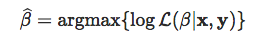The objective function here focuses (only) on the goodness of fit. But, usually, in econometrics, we believe something like non sunt multiplicanda entia sine necessitate ("entities are not to be multiplied without necessity"), the parsimony principle, simpler theories are preferable to more complex ones. So we want to penalize for models that are too complex.

This is not a bad idea. It is mentioned here and there in econometrics textbooks, but usually for model choice, not inference. Usually, we estimate parameters using maximum likelihood techniques, and them we use AIC or BIC to compare two models. Recall that Akaike (AIC) criteria are based on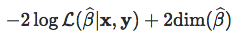We have on the left a measure for the goodness of fit, and on the right, a penalty increasing with the "complexity" of the model.

Very quickly, here, the complexity is the number of variates used. I will not enter into details about the concept of sparsity (and the true dimension of the problem), I will recommend reading the book by Martin Wainwright, Robert Tibshirani, and Trevor Hastie on that issue. But assume that we do not make a variable selection, we consider the regression on all covariates. Define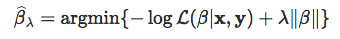for any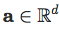One might say that the AIC could be written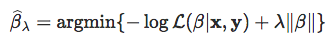And actually, this will be our objective function. More specifically, we will consider for some norm || . ||. I will not get back here on the motivation and the (theoretical) properties of those estimates (that will actually be discussed in the Summer School in Barcelona, in July), but, in this post, I want to discuss the numerical algorithm to solve such optimization problems, for|| . ||l1  (the Ridge regression) and for|| . ||l1  (the LASSO regression).

Normalization of the Covariates

The problem || ß ||of is that the norm should make sense, somehow. A small ß is with respect to the "dimension" of Xj's. So, the first step will be to consider linear transformations of all covariates Xj to get centered and scaled variables (with unit variance):

``````y = myocarde\$PRONO
X = myocarde[,1:7]
for(j in 1:7) X[,j] = (X[,j]-mean(X[,j]))/sd(X[,j])
X = as.matrix(X)``````

Ridge Regression (From Scratch)

Before running some code, recall that we want to solve something like: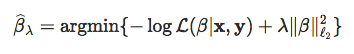In the case where we consider the log-likelihood of some Gaussian variable, we get the sum of the square of the residuals, and we can obtain an explicit solution. But not in the context of a logistic regression.

The heuristics about Ridge regression is the following graph. In the background, we can visualize the (two-dimensional) log-likelihood of the logistic regression, and the blue circle is the constraint we have if we rewrite the optimization problem as a constrained optimization problem :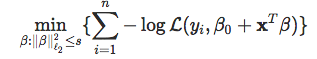can be written equivalently (it is a strictly convex problem)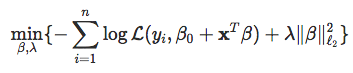Thus, the constrained maximum should lie in the blue disk

``````LogLik = function(bbeta){
b0=bbeta
beta=bbeta[-1]
sum(-y*log(1 + exp(-(b0+X%*%beta))) -
(1-y)*log(1 + exp(b0+X%*%beta)))}
u = seq(-4,4,length=251)
v = outer(u,u,function(x,y) LogLik(c(1,x,y)))
image(u,u,v,col=rev(heat.colors(25)))
u = seq(-1,1,length=251)
lines(u,sqrt(1-u^2),type="l",lwd=2,col="blue")
lines(u,-sqrt(1-u^2),type="l",lwd=2,col="blue")``````Let us consider the objective function, with the following code:

``````PennegLogLik = function(bbeta,lambda=0){
b0   = bbeta
beta = bbeta[-1]
-sum(-y*log(1 + exp(-(b0+X%*%beta))) - (1-y)*
log(1 + exp(b0+X%*%beta)))+lambda*sum(beta^2)
}``````

Why not try a standard optimization routine? In the very first post on that series, we did mention that using optimization routines was not clever since they were strongly relying on the starting point. But here, that is not the case.

``````lambda = 1
beta_init = lm(PRONO~.,data=myocarde)\$coefficients
vpar = matrix(NA,1000,8)
for(i in 1:1000){
vpar[i,] = optim(par = beta_init*rnorm(8,1,2),
function(x) PennegLogLik(x,lambda), method = "BFGS", control = list(abstol=1e-9))\$par}
par(mfrow=c(1,2))
plot(density(vpar[,2]),ylab="",xlab=names(myocarde))
plot(density(vpar[,3]),ylab="",xlab=names(myocarde))``````Clearly, even if we change the starting point, it looks like we converge towards the same value. That could be considered as the optimum.

The code to compute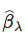would then be:

``````opt_ridge = function(lambda){
beta_init = lm(PRONO~.,data=myocarde)\$coefficients
logistic_opt = optim(par = beta_init*0, function(x) PennegLogLik(x,lambda),
method = "BFGS", control=list(abstol=1e-9))
logistic_opt\$par[-1]}``````

and we can visualize the evolution ofas a function of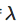``````v_lambda = c(exp(seq(-2,5,length=61)))
est_ridge = Vectorize(opt_ridge)(v_lambda)
library("RColorBrewer")
colrs = brewer.pal(7,"Set1")
plot(v_lambda,est_ridge[1,],col=colrs)
for(i in 2:7) lines(v_lambda,est_ridge[i,],col=colrs[i])``````At least it seems to make sense: we can observe the shrinkage asincreases (we'll get back to that later on).

Ridge, Using Netwon Raphson Algorithm

We've seen that we can also use Newton Raphson to solve this problem. Without the penalty term, the algorithm was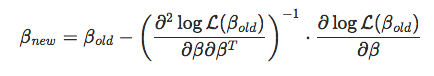whereand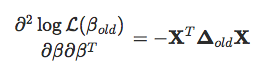where ∆old is the diagonal matrix with terms Pold(1-Pold) on the diagonal.

Thus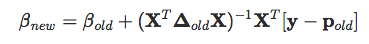that we can also write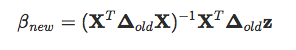where z=Xßold+(X^ToldX)^-1X^T[y-pold]. Here, on the penalized problem, we can easily prove that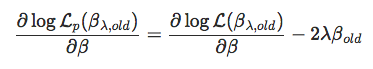while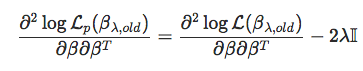Hence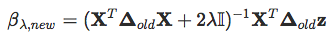The code is then:

``````Y = myocarde\$PRONO
X = myocarde[,1:7]
for(j in 1:7) X[,j] = (X[,j]-mean(X[,j]))/sd(X[,j])
X = as.matrix(X)
X = cbind(1,X)
colnames(X) = c("Inter",names(myocarde[,1:7]))
beta = as.matrix(lm(Y~0+X)\$coefficients,ncol=1)
for(s in 1:9){
pi = exp(X%*%beta[,s])/(1+exp(X%*%beta[,s]))
Delta = matrix(0,nrow(X),nrow(X));diag(Delta)=(pi*(1-pi))
z = X%*%beta[,s] + solve(Delta)%*%(Y-pi)
B = solve(t(X)%*%Delta%*%X+2*lambda*diag(ncol(X))) %*% (t(X)%*%Delta%*%z)
beta = cbind(beta,B)}
beta[,8:10]
[,1]        [,2]        [,3]
XInter  0.59619654  0.59619654  0.59619654
XFRCAR  0.09217848  0.09217848  0.09217848
XINCAR  0.77165707  0.77165707  0.77165707
XINSYS  0.69678521  0.69678521  0.69678521
XPRDIA -0.29575642 -0.29575642 -0.29575642
XPAPUL -0.23921101 -0.23921101 -0.23921101
XPVENT -0.33120792 -0.33120792 -0.33120792
XREPUL -0.84308972 -0.84308972 -0.84308972``````

Again, it seems that convergence is very fast.

And interestingly, with that algorithm, we can also derive the variance of the estimator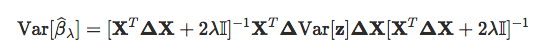where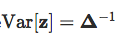The code to compute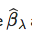as a function ofis then:

``````newton_ridge = function(lambda=1){
beta = as.matrix(lm(Y~0+X)\$coefficients,ncol=1)*runif(8)
for(s in 1:20){
pi = exp(X%*%beta[,s])/(1+exp(X%*%beta[,s]))
Delta = matrix(0,nrow(X),nrow(X));diag(Delta)=(pi*(1-pi))
z = X%*%beta[,s] + solve(Delta)%*%(Y-pi)
B = solve(t(X)%*%Delta%*%X+2*lambda*diag(ncol(X))) %*% (t(X)%*%Delta%*%z)
beta = cbind(beta,B)}
Varz = solve(Delta)
Varb = solve(t(X)%*%Delta%*%X+2*lambda*diag(ncol(X))) %*% t(X)%*% Delta %*% Varz %*%
Delta %*% X %*% solve(t(X)%*%Delta%*%X+2*lambda*diag(ncol(X)))
return(list(beta=beta[,ncol(beta)],sd=sqrt(diag(Varb))))}``````

We can visualize the evolution of(as a function of Lambda)

``````v_lambda=c(exp(seq(-2,5,length=61)))
est_ridge=Vectorize(function(x) newton_ridge(x)\$beta)(v_lambda)
library("RColorBrewer")
colrs=brewer.pal(7,"Set1")
plot(v_lambda,est_ridge[1,],col=colrs,type="l")
for(i in 2:7) lines(v_lambda,est_ridge[i,],col=colrs[i])``````and to get the evolution of the variance

``````v_lambda=c(exp(seq(-2,5,length=61)))
est_ridge=Vectorize(function(x) newton_ridge(x)\$sd)(v_lambda)
library("RColorBrewer")
colrs=brewer.pal(7,"Set1")
plot(v_lambda,est_ridge[1,],col=colrs,type="l")
for(i in 2:7) lines(v_lambda,est_ridge[i,],col=colrs[i],lwd=2)``````Recall that when Lambda=0 (on the left of the graphs),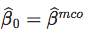(no penalty). Thus as Lambda increases (i) the bias increase (estimates tend to 0) (ii) the variances decrease.

Ridge, Using glmnet

As always, there are R functions available to run a ridge regression. Let's use the glmnet function, with Alpha=0

``````y = myocarde\$PRONO
X = myocarde[,1:7]
for(j in 1:7) X[,j] = (X[,j]-mean(X[,j]))/sd(X[,j])
X = as.matrix(X)
library(glmnet)
glm_ridge = glmnet(X, y, alpha=0)
plot(glm_ridge,xvar="lambda",col=colrs,lwd=2)``````as a function of the normThe l1 norm here, I don't know why. I don't know either why all graphs obtained with different optimization routines are so different... Maybe that will be for another post...

Ridge With Orthogonal Covariates

An interesting case is obtained when covariates are orthogonal. This can be obtained using a PCA of the covariates.

``````library(factoextra)
pca = princomp(X)
pca_X = get_pca_ind(pca)\$coord``````

Let's run a ridge regression on those (orthogonal) covariates

``````library(glmnet)
glm_ridge = glmnet(pca_X, y, alpha=0)
plot(glm_ridge,xvar="lambda",col=colrs,lwd=2)````````plot(glm_ridge,col=colrs,lwd=2)``We clearly observe the shrinkage of the parameters, in the sense that: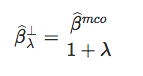Application

Let us try with our second set of data

``````df0 = df
df0\$y=as.numeric(df\$y)-1
plot_lambda = function(lambda){
m = apply(df0,2,mean)
s = apply(df0,2,sd)
for(j in 1:2) df0[,j] = (df0[,j]-m[j])/s[j]
reg = glmnet(cbind(df0\$x1,df0\$x2), df0\$y==1, alpha=0,lambda=lambda)
u = seq(0,1,length=101)
p = function(x,y){
xt = (x-m)/s
yt = (y-m)/s
predict(reg,newx=cbind(x1=xt,x2=yt),type='response')}
v = outer(u,u,p)
image(u,u,v,col=clr10,breaks=(0:10)/10)
points(df\$x1,df\$x2,pch=c(1,19)[1+z],cex=1.5)
}``````

We can try various values of Lambda:

``````reg = glmnet(cbind(df0\$x1,df0\$x2), df0\$y==1, alpha=0)
par(mfrow=c(1,2))
plot(reg,xvar="lambda",col=c("blue","red"),lwd=2)
abline(v=log(.2))
plot_lambda(.2)``````or

``````reg = glmnet(cbind(df0\$x1,df0\$x2), df0\$y==1, alpha=0)
par(mfrow=c(1,2))
plot(reg,xvar="lambda",col=c("blue","red"),lwd=2)
abline(v=log(1.2))
plot_lambda(1.2)``````The next step is to change the norm of the penalty, with the l1 norm (to be continued...).

Topics:
big data ,data analysis ,regression analysis ,r

Comment (0)

Save
{{ articles.views | formatCount}} Views

Published at DZone with permission of

Opinions expressed by DZone contributors are their own.